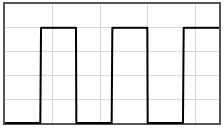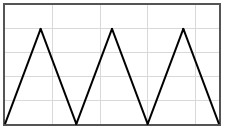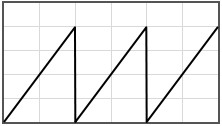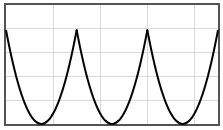## Bloch waves in 1-D

It is instructive to consider a one-dimensional crystal at this point since the dispersion relation $E(\vec{k})$ can be readily calculated numerically in one-dimension. Consider an electron moving in a periodic potential $V(x)$. The period of the potential is $a$, $V(x+a) = V(x)$. The Schrödinger equation for this case is,

$\begin{equation} -\frac{\hbar^2}{2m}\frac{d^2\psi}{dx^2}+V(x)\psi =E\psi . \end{equation}$

Quantum mechanically, the electron moves as a wave through the potential. Due to the diffraction of these waves, there are bands of energies where the electron is allowed to propagate through the potential and bands of energies where no propagating solutions are possible. The Bloch theorem states that the propagating states have the form,

$\begin{equation} \psi = e^{ikx}u_k(x). \end{equation}$

where $k$ is the wavenumber and $u_k(x)$ is a periodic function with periodicity $a$.

There is a left moving Bloch wave $\psi_-=e^{-ikx}u_{k-}$ and a right moving Bloch wave $\psi_+=e^{ikx}u_{k+}$ for every energy. The following form calculates the Bloch waves for a potential $V(x)$ that is specified in the interval between $0$ and $a$. A discussion of the calculation can be found below the form.

 $V(x)$[eV] $x/a$
 The 1-D Potential Energy E = [eV] Mass m = [kg] Lattice constant a  = [m] Potential V(x)  = [eV]
 $\psi_+$ $x/a$ $\psi_-$ $x/a$
 $u_{k+}$ $x/a$ $u_{k-}$ $x/a$ $e^{ik\xi}$ $x/a$ $e^{-ik\xi}$ $x/a$ $\psi^*\psi$ $x/a$ $\frac{2ma}{\hbar}v_k$ $x/a$
 $\psi_1$ $\psi_2$ $x/a$ $x/a$

Data:

Standard mathematical functions abs(x), acos(x), asin(x), atan(x), cos(x), exp(x), log(x), pi = 3.141592653589793, pow(x,y) = xy, round(x), sin(x), sqrt(x) tan(x) can be used in the form. In addition, the Heaviside step function H(x) can be used. Multiplication must be specified with a '*' symbol, 3*cos(x) not 3cos(x). Powers are specified with the 'pow' function: x² is pow(x,2) not x^2.

Some potentials that can be pasted into the form are given below.### Solving the Schrödiger equation for a periodic potential in 1-D

 W. Magnus and S. Winkler, Hill's Equation, Dover 1966.